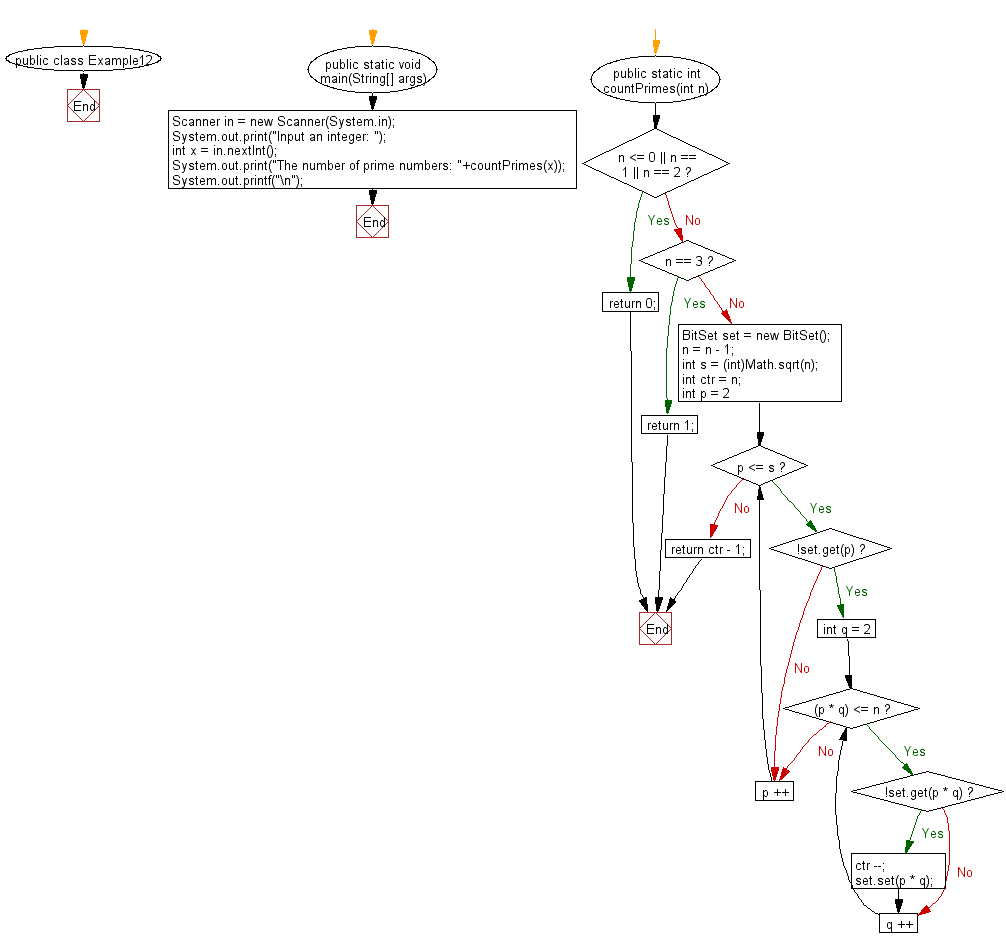﻿ Java Math Exercises: Count the number of prime numbers less than a given positive number - w3resource# Java Math Exercises: Count the number of prime numbers less than a given positive number

## Java Math Exercises: Exercise-12 with Solution

Write a Java program to count the number of prime numbers less than a given positive number.

Sample Solution:

Java Code:

``````import java.util.*;
public class Example12 {
public static void main(String[] args)
{
Scanner in = new Scanner(System.in);
System.out.print("Input an integer: ");
int x = in.nextInt();
System.out.print("The number of prime numbers: "+countPrimes(x));
System.out.printf("\n");
}

public static int countPrimes(int n)
{
if(n <= 0 || n == 1 || n == 2)
return 0;
else if(n == 3)
return 1;
BitSet set = new BitSet();
n = n - 1;
int s = (int)Math.sqrt(n);
int ctr = n;
for(int p = 2; p <= s; p ++)
{
if(!set.get(p))
{
for(int q = 2; (p * q) <= n; q ++)
{
if(!set.get(p * q))
{
ctr --;
set.set(p * q);
}
}
}
}
return ctr - 1;
}
}
``````

Sample Output:

```Input an integer: 25
The number of prime numbers: 9
```

Flowchart:Java Code Editor:

What is the difficulty level of this exercise?

﻿

## Java: Tips of the Day

Parsing dates:

```import java.io.*;
import java.util.*;
import java.text.*;

String s = "2001/09/23 14:39";

SimpleDateFormat formatter = new SimpleDateFormat ("yyyy/MM/dd H:mm");
Date d = formatter.parse(s, new ParsePosition(0));
```